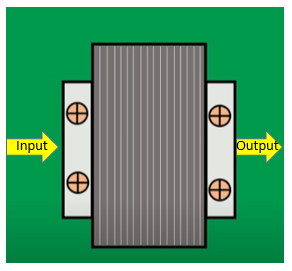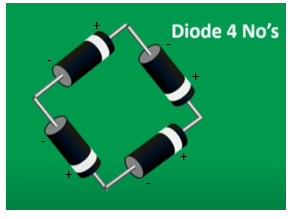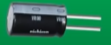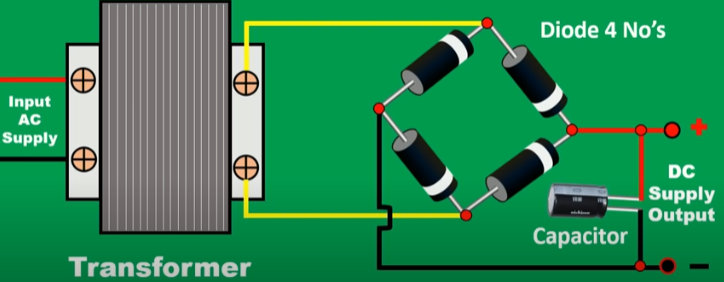# How to Convert AC to DC using Diodes

The process of converting AC to DC is known as Rectification. The AC-DC converters are mostly used in power electronic applications where the power input is a 50 Hz or 60 Hz sine-wave AC voltage that needs power conversion for a DC output.

Each and every appliance at our house works on Direct current (DC). However, we get Alternating current (AC) from the power generation plants via transmission lines. Hence, in all such cases we need AC-DC converter circuits to convert the AC to DC.

Here in this article, we will study the circuit design and operation of an AC to DC converter.

Alternating Current (AC):  The current that changes its direction periodically is called an alternating current (AC). It flows forward and backward. It has non-zero frequency. It is produced by AC generator, dynamo, etc.

Alternating Current (AC)

Direct Current (DC) :  The current that always flows in the same direction in a conductor is called direct current (DC). It doesn’t change its magnitude and polarity. It has zero frequency. It is produced by cells, battery, DC generator etc.

Direct Current (DC)

### Components Needed

1. 1 Capacitor
2. 1 Step Down Transformer
3. 4 Diodes

### Steps to make an AC to DC Converter

Step-1 (Stepping down the Voltage)

In step 1, take a Step Down Transformer. Normally the Input AC is 240 Volt. So to get the required DC output voltage which is less than the input we need a step-down transformer.Step-down Transformer

Step-2 (AC-DC Converter Circuit)

Here, we will use a full wave bridge rectifier circuit that consists of  four diodes D1 , D2,Dand D4, which are connected to form a bridge like structure.

So in this step, connect the 4 Diodes as shown in picture below to form a bridge like structure.Step-3

Now, in the third step, connect the capacitor to the circuit as shown in the diagram below.CapacitorFull wave Bridge Rectifier (AC-DC Converter) Circuit

The AC to DC converter is ready now. You can carry on your experiments and check the output.